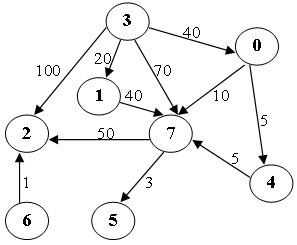# 6-17 Shortest Path  (25point(s))

Write a program to find the weighted shortest distances from any vertex to a given source vertex in a digraph. If there is more than one minimum path from v to w, a path with the fewest number of edges is chosen. It is guaranteed that all the weights are positive and such a path is unique for any vertex.

### Format of functions:

void ShortestDist( MGraph Graph, int dist[], int path[], Vertex S );

where MGraph is defined as the following:

typedef struct GNode *PtrToGNode;
struct GNode{
int Nv;
int Ne;
WeightType G[MaxVertexNum][MaxVertexNum];
};
typedef PtrToGNode MGraph;

The shortest distance from V to the source S is supposed to be stored in dist[V]. If V cannot be reached from S, store -1 instead. If W is the vertex being visited right before V along the shortest path from S to V, then path[V]=W. If V cannot be reached from Spath[V]=-1, and we have path[S]=-1.

### Sample program of judge:

#include <stdio.h>
#include <stdlib.h>

typedef enum {false, true} bool;
#define INFINITY 1000000
#define MaxVertexNum 10  /* maximum number of vertices */
typedef int Vertex;      /* vertices are numbered from 0 to MaxVertexNum-1 */
typedef int WeightType;

typedef struct GNode *PtrToGNode;
struct GNode{
int Nv;
int Ne;
WeightType G[MaxVertexNum][MaxVertexNum];
};
typedef PtrToGNode MGraph;

MGraph ReadG(); /* details omitted */

void ShortestDist( MGraph Graph, int dist[], int path[], Vertex S );

int main()
{
int dist[MaxVertexNum], path[MaxVertexNum];
Vertex S, V;
MGraph G = ReadG();

scanf("%d", &S);
ShortestDist( G, dist, path, S );

for ( V=0; V<G->Nv; V++ )
printf("%d ", dist[V]);
printf("\n");
for ( V=0; V<G->Nv; V++ )
printf("%d ", path[V]);
printf("\n");

return 0;
}

/* Your function will be put here */


### Sample Input (for the graph shown in the figure):8 11
0 4 5
0 7 10
1 7 40
3 0 40
3 1 20
3 2 100
3 7 70
4 7 5
6 2 1
7 5 3
7 2 50
3

### Sample Output:

40 20 100 0 45 53 -1 50
3 3 3 -1 0 7 -1 0

### Example:

void ShortestDist( MGraph Graph, int dist[], int path[], Vertex S )
{
int visited[MaxVertexNum];
for(int i = 0; i < Graph->Nv; i++) {
dist[i] = path[i] = -1;
visited[i] = false;
}
dist[S] = 0;
path[S] = -1;
for(int h = 0; h < Graph->Nv; h++) {
int min = -1;
for(int k = 0; k < Graph->Nv; k++) {
if(!visited[k] && (dist[k] > -1)) {
if((min == -1) || (dist[k] < dist[min])) {
min = k;
}
}
}
if(min == -1) return ;
visited[min] = true;
for(int i = 0; i < Graph->Nv; i++) {
if(Graph->G[min][i] < INFINITY)
if((dist[i] == -1) || (dist[i]>Graph->G[min][i]+dist[min])) {
dist[i] = Graph->G[min][i] + dist[min];
path[i] = min;
}
}
}
}

10-31374
11-0615611-05282
02-252147
12-0953
01-1413
11-23279
11-2791
12-07650
10-30328
09-112392
11-144228
06-14313
04-14807
07-261505
12-15632
05-23331
04-171万+
06-19181
©️2020 CSDN 皮肤主题: 大白 设计师:CSDN官方博客